# Check if each element of the given array is the product of exactly K prime numbers

Given an array of numbersand the value of, check if each numbercan be expressed as the product of exactlyprime numbers. For every element of the array print ‘YES’ if the condition is satisfied, else print ‘NO’.

Note: Repeating prime numbers may also be considered. For example, if k = 2, then n = 4 (=2*2) is a valid input.

Let’s consider the number 36. 36 can be factorised as 2*2*3*3. Hence, it is the product of 4 prime numbers. If k value is 4, then the output should be YES. For other values of k, output should be NO.

More Examples:

Input:  arr[] = {30, 8, 12}, K = 3
Output: YES, YES, YES
30 = 2*3*5
8 = 2*2*2
12 = 2*3*2

Input: arr[] = {30, 16, 32}, k = 5
Output: NO, NO, YES
Only 32 can be represented as product of
5 prime numbers.


## Recommended: Please try your approach on {IDE} first, before moving on to the solution.

In this article, we shall check if the given number(s) can be expressed as the product of exactly k prime numbers. The underlying concept that we shall we be using is simply a variation of the Sieve of Eratosthenes.

Recommended: How to construct the Sieve of Eratosthenes

Key Difference: In the Sieve, instead of storing binary values (0 if number not prime, 1 if the number is prime), we can store how many (repeating) prime factors make up that that number instead. This modification is done during its construction.

The generalised procedure to create this modified sieve is as follows:

1. Create an array full of 0’s to store the list of consecutive integers (2, 3, 4 … 10^6).
2. Let the value of i be set to 2 initially. This is our first prime number.
3. Loop through all the multiples of i (2*i, 3*i … till 10^6) by storing its value as j. Proceed with steps 3 and 4.
4. Calculate the number of times j can be factorised using i and store the result into the variable count.
5. When the number j cannot be further factorised using i, increment the value of Sieve[j] by the count value.
6. Finally, find the next prime number greater than i in the list of integers. If there is no such number then terminate the process. Else, follow from step 2 again.

Explanation with example:

STEP 1:

The initialised empty sieve array looks as illustrated below. For simplicity’s sake let’s only concentrate on indices 2 through 12. The values stored initially is 0 for all indices.

Now, the first prime number that we will take is 2. This is the value of i.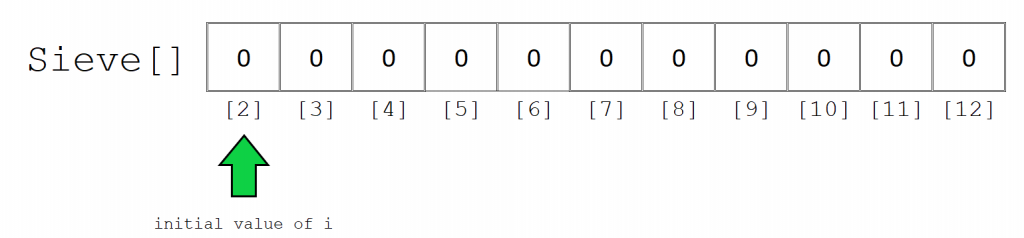STEP 2:

Initialise variable j to hold the value of every subsequent multiple of i starting from 2*i, which in this case is 4.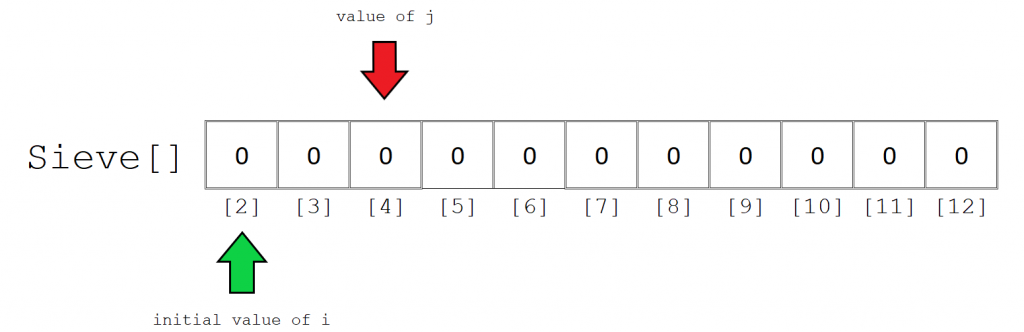STEP 3:

The third step involves the computation of the prime factorisation of j. More specifically, we are just interested in counting the number of occurrences of i when you factorise j.

The computation procedure is simple. Just divide the value of j with i until you get a number that is not divisible by i. Here, 4 can be divided by 2 twice. 4/2 yields 2 and 2/2 yields 1, which is not divisible by 2 and the loop stops. Hence, we update the value of Sieve with the value of the count variable, which is 2.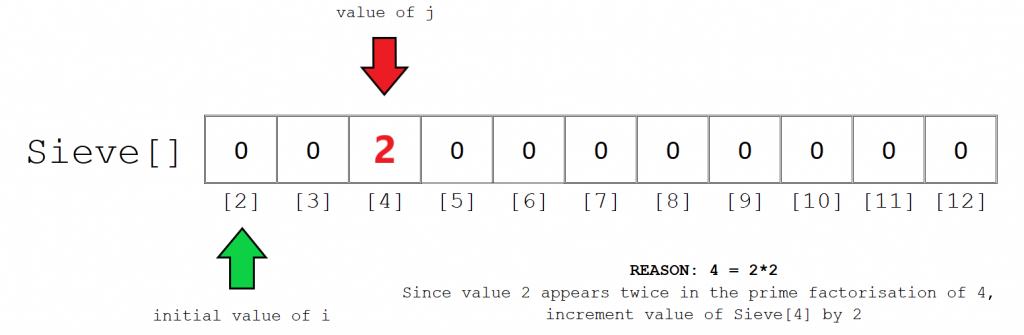STEP 4:

We can proceed with the other elements in a similar fashion. Next, the value of j is 6. 6 can only be divided by 2 once. Hence the value of Sieve is 1.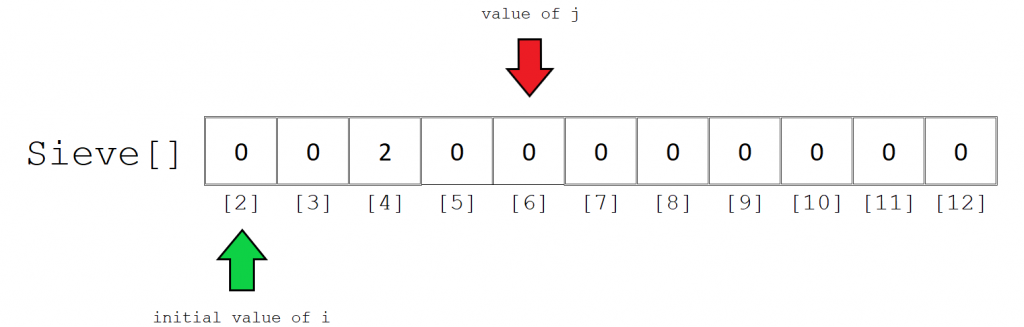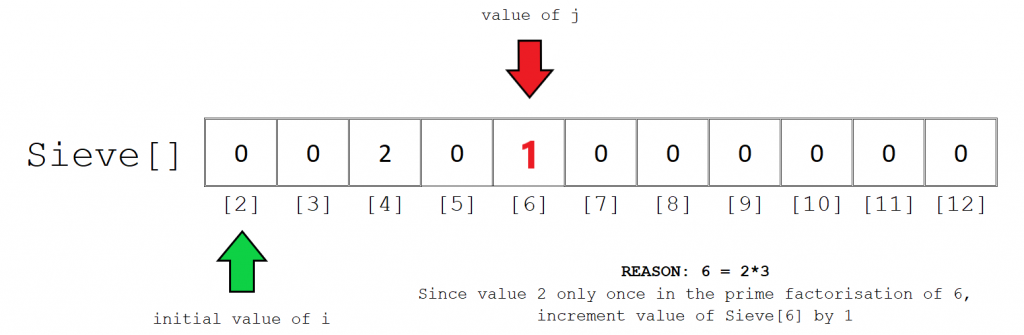The final computed Sieve array should look something like this. Note that any index storing the value 0 represents a number that is not the product of 2 or more prime numbers. This includes all prime numbers, 0 and 1.

The second thing to note is that we need to only check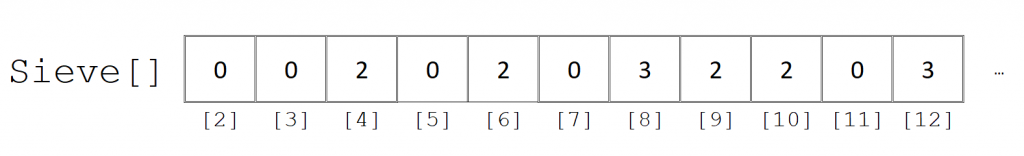Below is the implementation of the above approach:

## C++

 // C++ program to check if each element of  // the given array is a product of exactly  // K prime factors     #include  #define MAX 1000000  using namespace std;     // initialise the global sieve array  int Sieve[MAX] = { 0 };     // Function to generate Sieve  void constructSieve()  {      // NOTE: k value is necessarily more than 1      // hence, 0, 1 and any prime number cannot be      // represented as product of      // two or more prime numbers         for (int i = 2; i <= MAX; i++) {          if (Sieve[i] == 0) {              for (int j = 2 * i; j <= MAX; j += i) {                  int temp = j;                  while (temp > 1 && temp % i == 0) {                      Sieve[j]++;                      temp = temp / i;                  }              }          }      }  }     // Function to check if each number of array  // satisfies the given condition  void checkElements(int A[], int n, int k)  {      for (int i = 0; i < n; i++) {          if (Sieve[A[i]] == k) {              cout << "YES\n";          }          else {              cout << "NO\n";          }      }  }     // Driver Code  int main()  {      // first construct the sieve      constructSieve();         int k = 3;      int A[] = { 12, 36, 42, 72 };      int n = sizeof(A) / sizeof(int);         checkElements(A, n, k);         return 0;  }

## Java

 // Java program to check if each element of  // the given array is a product of exactly  // K prime factors  import java.util.*;     class GFG   {         static int MAX = 1000000;         // initialise the global sieve array      static int[] Sieve = new int[MAX+1];         // Function to generate Sieve      static void constructSieve()       {          // NOTE: k value is necessarily more than 1          // hence, 0, 1 and any prime number cannot be          // represented as product of          // two or more prime numbers             for (int i = 2; i <= MAX; i++)           {              if (Sieve[i] == 0)               {                  for (int j = 2 * i; j <= MAX; j += i)                  {                      int temp = j;                      while (temp > 1 && temp % i == 0)                      {                          Sieve[j]++;                          temp = temp / i;                      }                  }              }          }      }         // Function to check if each number of array      // satisfies the given condition      static void checkElements(int A[], int n, int k)       {          for (int i = 0; i < n; i++)           {              if (Sieve[A[i]] == k)              {                  System.out.println("YES");              }               else             {                  System.out.println("No");              }             }      }         // Driver Code      public static void main(String[] args)      {          // first construct the sieve          constructSieve();             int k = 3;          int A[] = {12, 36, 42, 72};          int n = A.length;             checkElements(A, n, k);      }  }     // This code contributed by Rajput-Ji

## Python3

 # Python3 program to check if each element of   # the given array is a product of exactly   # K prime factors      MAX = 1000000    # initialise the global sieve array   Sieve = *(MAX + 1)      # Function to generate Sieve   def constructSieve() :         # NOTE: k value is necessarily more than 1       # hence, 0, 1 and any prime number cannot be       # represented as product of       # two or more prime numbers          for i in range(2, MAX + 1) :          if (Sieve[i] == 0) :              for j in range(2*i, MAX + 1, i) :                  temp = j;                   while (temp > 1 and temp % i == 0) :                       Sieve[j] += 1;                       temp = temp // i;                 # Function to check if each number of array   # satisfies the given condition   def checkElements(A, n, k) :          for i in range(n) :           if (Sieve[A[i]] == k) :              print("YES");                         else :              print("NO");      # Driver Code   if __name__ == "__main__" :         # first construct the sieve       constructSieve();          k = 3;       A = [ 12, 36, 42, 72 ];       n = len(A);          checkElements(A, n, k);          # This code is contributed by AnkitRai01

## C#

 // C# program to check if each element of  // the given array is a product of exactly  // K prime factors  using System;     class GFG   {         static int MAX = 1000000;         // initialise the global sieve array      static int[] Sieve = new int[MAX+1];         // Function to generate Sieve      static void constructSieve()       {          // NOTE: k value is necessarily more than 1          // hence, 0, 1 and any prime number cannot be          // represented as product of          // two or more prime numbers             for (int i = 2; i <= MAX; i++)           {              if (Sieve[i] == 0)               {                  for (int j = 2 * i; j <= MAX; j += i)                  {                      int temp = j;                      while (temp > 1 && temp % i == 0)                      {                          Sieve[j]++;                          temp = temp / i;                      }                  }              }          }      }         // Function to check if each number of array      // satisfies the given condition      static void checkElements(int []A, int n, int k)       {          for (int i = 0; i < n; i++)           {              if (Sieve[A[i]] == k)              {                  Console.WriteLine("YES");              }               else             {                  Console.WriteLine("No");              }             }      }         // Driver Code      public static void Main()      {          // first construct the sieve          constructSieve();             int k = 3;          int []A = {12, 36, 42, 72};          int n = A.Length;             checkElements(A, n, k);      }  }     // This code contributed by anuj_67...

Output:

YES
NO
YES
NO


Time Complexity: O(n*log(logn))
Space Complexity: O(MAX), where MAX is 106.

Attention reader! Don’t stop learning now. Get hold of all the important DSA concepts with the DSA Self Paced Course at a student-friendly price and become industry ready.

My Personal Notes arrow_drop_upCheck out this Author's contributed articles.

If you like GeeksforGeeks and would like to contribute, you can also write an article using contribute.geeksforgeeks.org or mail your article to contribute@geeksforgeeks.org. See your article appearing on the GeeksforGeeks main page and help other Geeks.

Please Improve this article if you find anything incorrect by clicking on the "Improve Article" button below.

Improved By : Rajput-Ji, vt_m, AnkitRai01